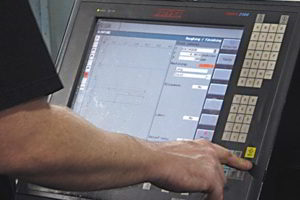# Sinumerik 840D System Variable \$AA_IM for Axis Current PositionSinumerik 840D System Variable \$AA_IM for Axis Current Position

Siemens Sinumerik 840D gives cnc machinist the utmost flexibility to program the CNC machine the way he likes. You can create your own variables. You can access the Sinumerik 840D system variables.

Here is how to access axis current positions through Sinumerik 840D system variables .

## Sinumerik 840D System Variable \$AA_IM

\$AA_IM tells us current axis value in the machine coordinate system MCS.

### Example

`N20 IF \$AA_IM [X] <= 15 GOTOF ERROR_202`

The line above checks if the x axis slide is under 15 then go to an error (there might be a danger of some collision). It must be clear that this variable gives the values in machine coordinate system MCS.

## Sinumerik 840D System Variable \$AA_IW

If you want to take the values that are calculated through workpiece coordinate system then try AA_IW[axis] which reads actual value in WCS

### Example

`N20 IF \$AA_IW [X] <= 15 GOTOF ERROR_202`

You can even try this

`N20 MSG ("X-position" << \$AA_IW[X] << "Check!")`

The above code will show the message on the machine control panel with x-axis current value (MCS).